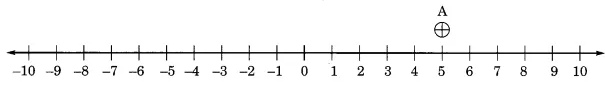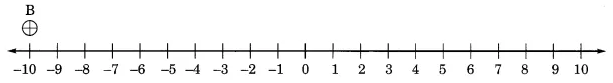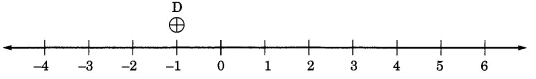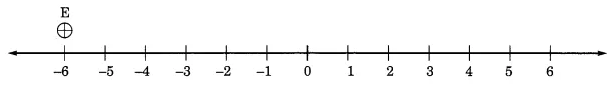Q

# Represent the following numbers on a number line: (a) plus 5

3.     Represent the following numbers on a number line :

(a) + 5

(b) – 10

(c) + 8

(d) – 1

(e) – 6

Views

Draw a straight line and place a mark on it at a distance of the 1cm unit.

(a) Here A indicates the position of(b) Here B indicates the position of(c) Here C represents the position of(d) Here D represents the(e) Here E represents theExams
Articles
Questions